如何用 QUnit 来测试 JavaScript 代码

【福利】CTO直接拍板说好用的SDK全在这里了！0元配齐，全部免费！>>>QUnit是一套由jQuery团队开发的，非常强大的用于对JavaScript进行单元测试的框架。本文将介绍什么是QUnit，以及为何要关心代码测试。

Qunit是一款强大的用于帮助调试代码的，JavaScript单元测试框架。QUnit由jQuery团队成员编写，是jQuery的官方测试套件，不仅如此，QUnit还可以测试任何常规JavaScript代码，甚至可以通过一些像Rhino或者V8这样的JavaScript引擎，测试服务端JavaScript代码。

"在计算机编程中，单元测试（又称为模块测试）是针对程序模块(软件设计的最小单位)来进行正确性检验的测试工作。程序单元是应用的最小可测试部件。在过程化编程中，一个单元就是单个程序、函数、过程等；对于面向对象编程，最小单元就是方法，包括基类（超类）、抽象类、或者派生类（子类）中的方法。"——引自维基百科。

<!DOCTYPE html>
<html>
<title>QUnit Test Suite</title>
<link rel="stylesheet" href="http://github.com/jquery/qunit/raw/master/qunit/qunit.css" mce_href="http://github.com/jquery/qunit/raw/master/qunit/qunit.css" type="text/css" media="screen">
<mce:script type="text/javascript" src="http://github.com/jquery/qunit/raw/master/qunit/qunit.js" mce_src="http://github.com/jquery/qunit/raw/master/qunit/qunit.js"></mce:script>
<!-- Your project file goes here -->
<mce:script type="text/javascript" src="myProject.js" mce_src="myProject.js"></mce:script>
<!-- Your tests file goes here -->
<mce:script type="text/javascript" src="myTests.js" mce_src="myTests.js"></mce:script>
<body>
<h1 id="qunit-header">QUnit Test Suite</h1>
<h2 id="qunit-banner"></h2>
<div id="qunit-testrunner-toolbar"></div>
<h2 id="qunit-userAgent"></h2>
<ol id="qunit-tests"></ol>
</body>
</html>

“断言是一个命题，预测你的代码的返回结果。如果预测是假的，断言失败，你就知道出了问题。”

// Let's test this function
function isEven(val) {
return val % 2 === 0;
}
test('isEven()', function() {
ok(isEven(0), 'Zero is an even number');
ok(isEven(2), 'So is two');
ok(isEven(-4), 'So is negative four');
ok(!isEven(1), 'One is not an even number');
ok(!isEven(-7), 'Neither is negative seven');
})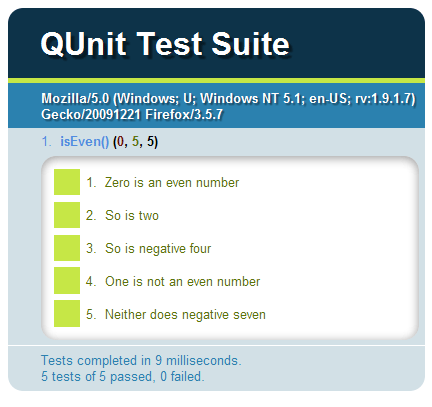// Let's test this function
function isEven(val) {
return val % 2 === 0;
}
test('isEven()', function() {
ok(isEven(0), 'Zero is an even number');
ok(isEven(2), 'So is two');
ok(isEven(-4), 'So is negative four');
ok(!isEven(1), 'One is not an even number');
ok(!isEven(-7), 'Neither does negative seven');
// Fails
ok(isEven(3), 'Three is an even number');
})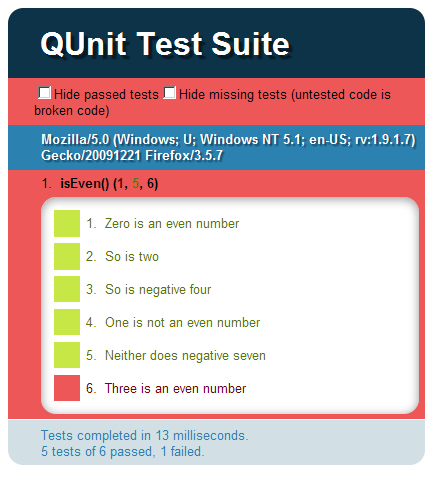ok()不仅是QUnit提供的唯一断言， 当在测试你的项目时，还会有一些非常有用的其他类型的断言:

test('assertions', function() {
ok( 1 == 1, 'one equals one');
})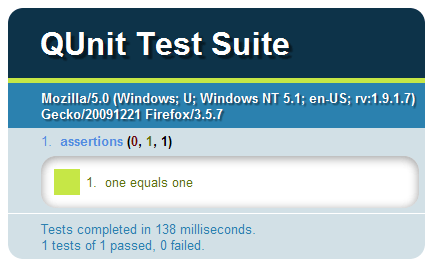test('assertions', function() {
equals( 1, 1, 'one equals one');
})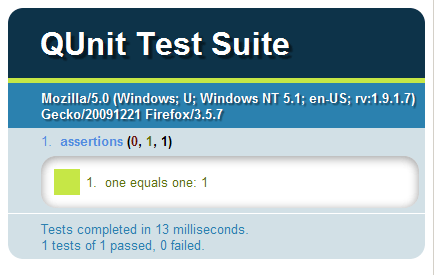test('assertions', function() {
equals( 2, 1, 'one equals one');
})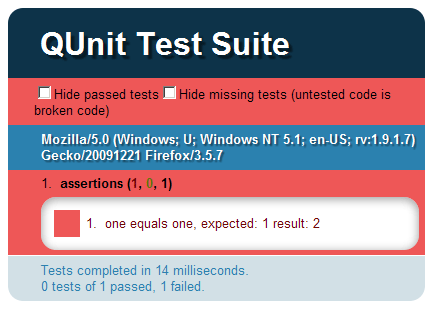test('test', function() {
equals( {}, {}, 'fails, these are different objects');
equals( {a: 1}, {a: 1} , 'fails');
equals( [], [], 'fails, there are different arrays');
equals( , , 'fails');
})

test('test', function() {
same( {}, {}, 'passes, objects have the same content');
same( {a: 1}, {a: 1} , 'passes');
same( [], [], 'passes, arrays have the same content');
same( , , 'passes');
})

test('test', function() {
equals( 0, false, 'true');
same( 0, false, 'false');
equals( null, undefined, 'true');
same( null, undefined, 'false');
})

module('Module A');
test('a test', function() {});
test('an another test', function() {});
module('Module B');
test('a test', function() {});
test('an another test', function() {});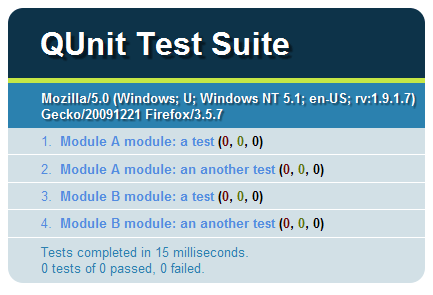test('asynchronous test', function() {
setTimeout(function() {
ok(true);
}, 100)
})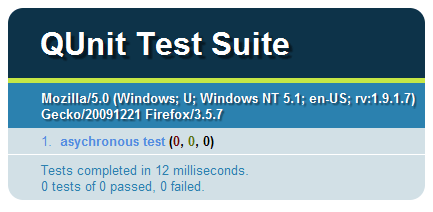test('asynchronous test', function() {
// Pause the test first
stop();

setTimeout(function() {
ok(true);

// After the assertion has been called,
// continue the test
start();
}, 100)
})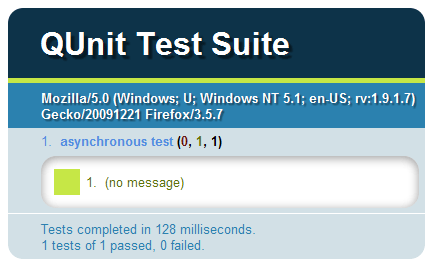asyncTest('asynchronous test', function() {
// The test is automatically paused

setTimeout(function() {
ok(true);

// After the assertion has been called,
// continue the test
start();
}, 100)
})// A custom function
function ajax(successCallback) {
\$.ajax({
url: 'server.php',
success: successCallback
});
}

test('asynchronous test', function() {
// Pause the test, and fail it if start() isn't called after one second
stop(1000);

ajax(function() {
// ...asynchronous assertions

start();
})
})

// A custom function
function ajax(successCallback) {
\$.ajax({
url: 'server.php',
success: successCallback
});
}

test('asynchronous test', function() {
// Pause the test
stop();

ajax(function() {
// ...asynchronous assertions
})

ajax(function() {
// ...asynchronous assertions
})

setTimeout(function() {
start();
}, 2000);
})

// A custom function
function ajax(successCallback) {
\$.ajax({
url: 'server.php',
success: successCallback
});
}

test('asynchronous test', function() {
// Pause the test
stop();

// Tell QUnit that you expect three assertions to run
expect(3);

ajax(function() {
ok(true);
})

ajax(function() {
ok(true);
ok(true);
})

setTimeout(function() {
start();
}, 2000);
})

// A custom function
function ajax(successCallback) {
\$.ajax({
url: 'server.php',
success: successCallback
});
}

// Tell QUnit that you expect three assertion to run
test('asynchronous test', 3, function() {
// Pause the test
stop();

ajax(function() {
ok(true);
})

ajax(function() {
ok(true);
ok(true);
})

setTimeout(function() {
start();
}, 2000);
})

0

0

0

0